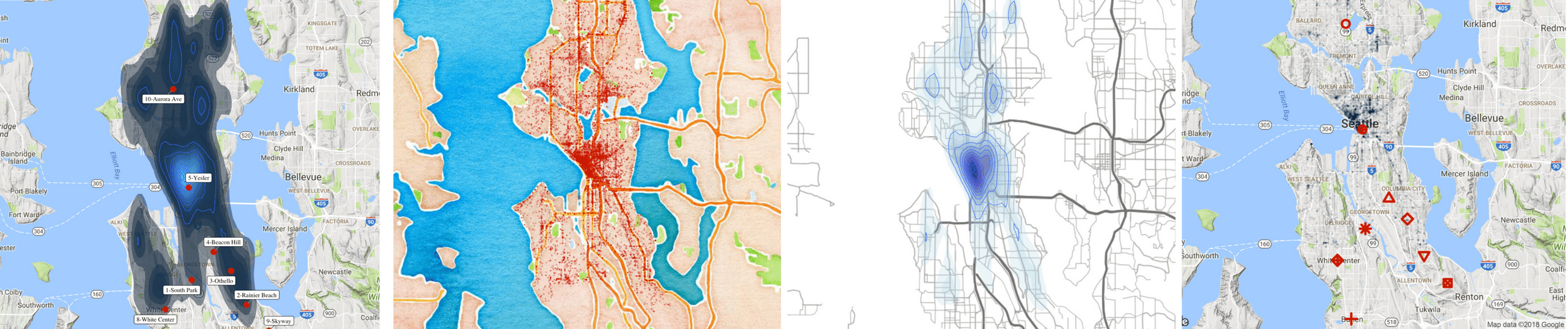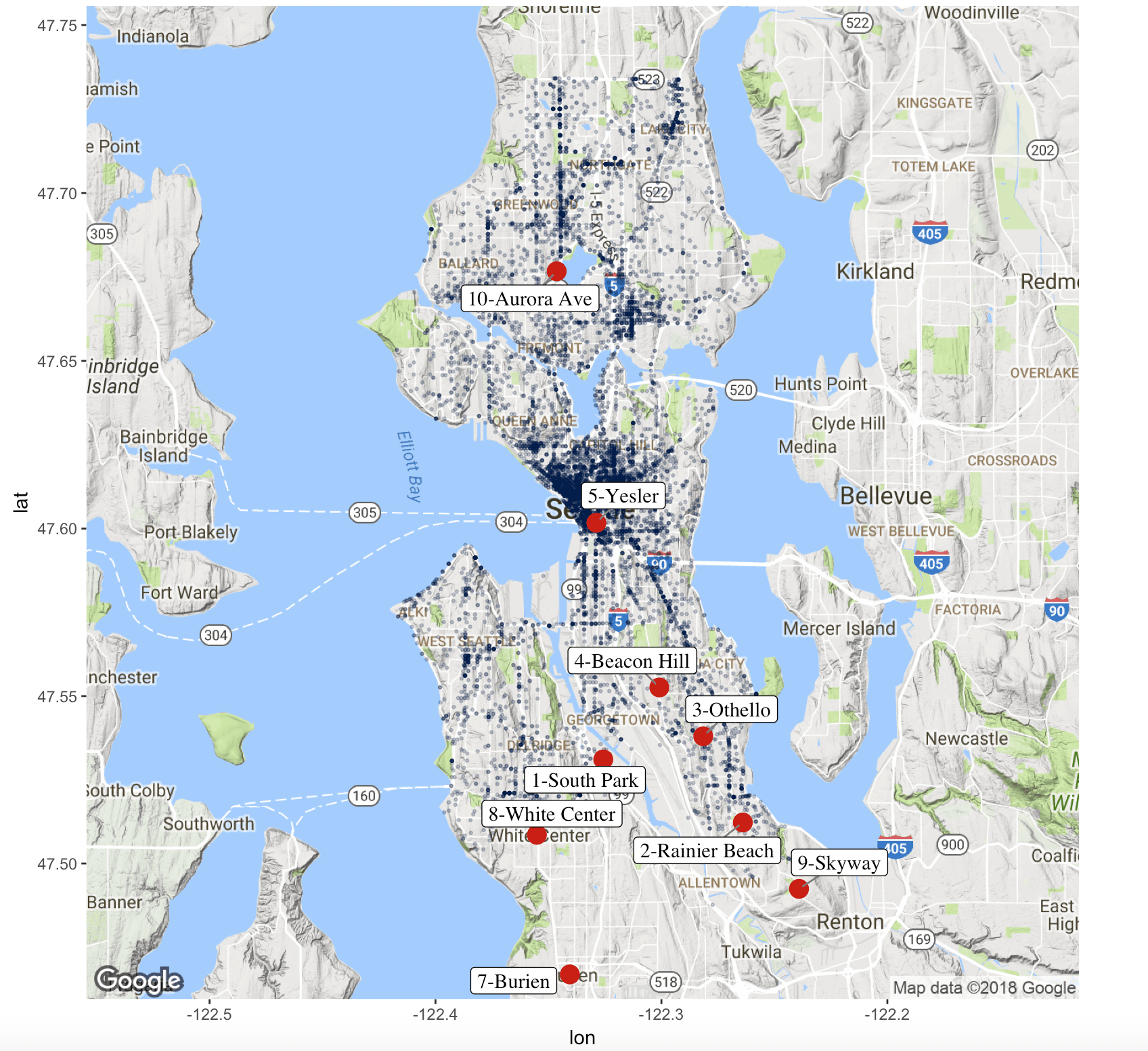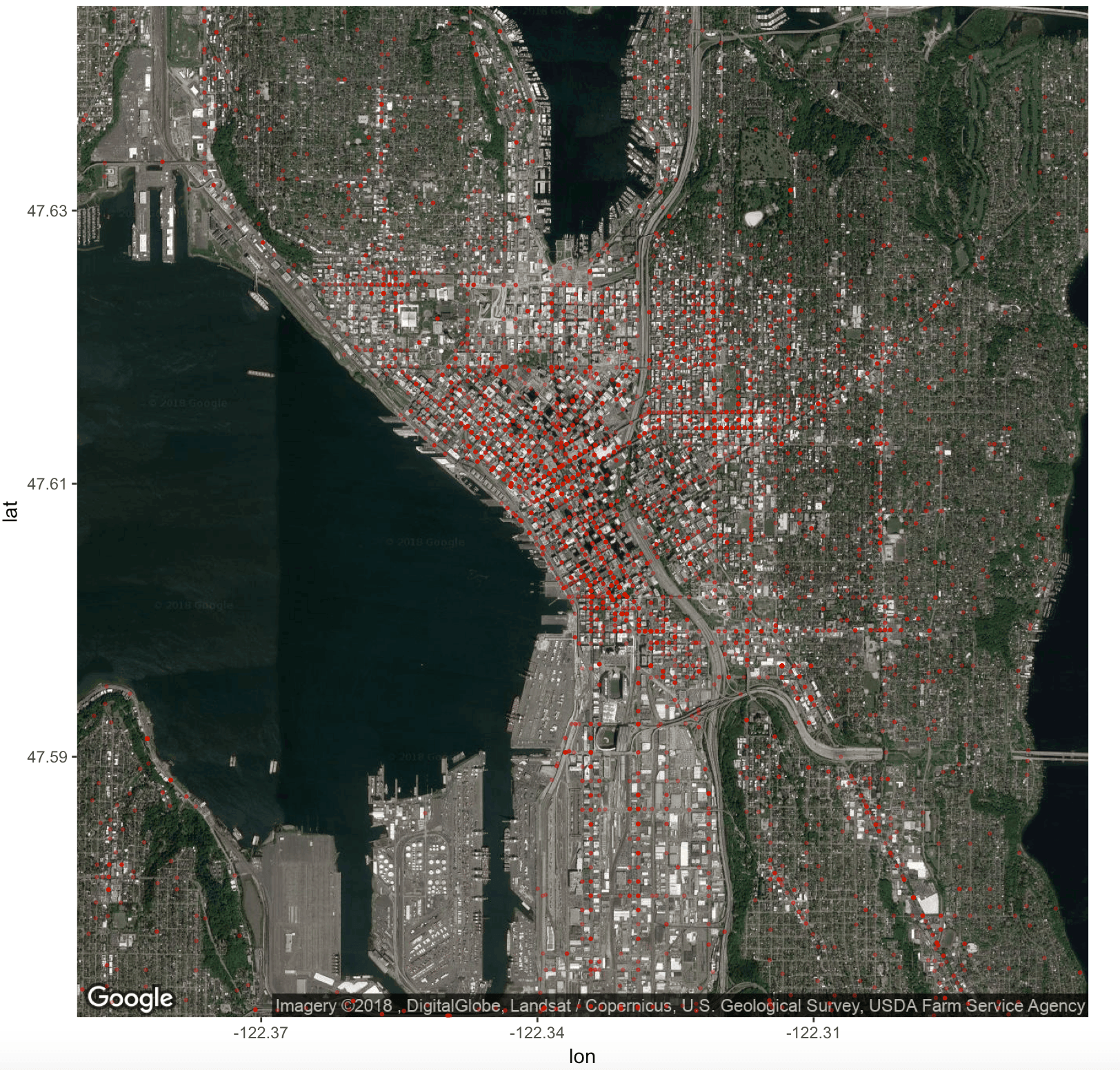Want to share your content on R-bloggers? click here if you have a blog, or here if you don't.In my previous tutorial we created heatmaps of Seattle 911 call volume by various time periods and groupings.   The analysis was based on a dataset which provides Seattle 911 call metadata.  It’s available as part of the data.gov open data project.  The results were really neat, but it got me thinking – we have latitude and longitude within the dataset, so why don’t we create some geographical graphs?

Y’all know I’m a sucker for a beautiful data visualizations and I know just the package to do this: GGMAP!   The ggmap package extends the ever-amazing ggplot core features to allow for spatial and geograhic visualization.

So let’s get going!

### 1) Set Up R

In terms of setting up the R working environment, we have a couple of options open to us.  We can use something like R Studio for a local analytics on our personal computer.  Or we can use a hosted, multi-language collaboration environment like Watson Studio.   In this tutorial we are going to be using IBM Cloud Lite account and Watson Studio to host our data set and and run the R analysis.  If you are going to use an alternative R installation like R studio, skip to step 2.B) Deploy Watson Studio from the catalog.  Note this was previously called Data Science Experience.

Select the “Lite” plan and hit “Create”.

You will then be taken to new screen where you can click “Get started”.  This will redirect you to the Watson Studio UI.C) Create a New Project – It’s best to start by creating a project so that you can store the R notebook and other assets together logically (models, data connections etc).  Create the project.  If this is your first project, you will also need to create an object storage service to store your data.  This is free and just a few clicks.D) Create a New Notebook –  Notebooks are a cool way of writing code, because they allow you to weave in the execution of code and display of content and at the same time.

Select “Assets”.  Select “New Notebook”.  Set the proper paramaters: name, description, project etc.Ensure you select an R environment as the notebook environment.  Click createFor each step below, the instructions are: Create a new cell.  Enter the code below.  Run the code by pressing the top nav button “run cell” which looks like a right arrow.

Note: If you need to close and reopen your notebook, please make sure to click the edit button in the upper right so that you can interact with the notebook and run the code.

### 2) Install and load packages

R packages contain a grouping of R data functions and code that can be used to perform your analysis. We need to install and load them in your environment so that we can call upon them later.  If you are in Watson Studio, enter the following code into a new cell, highlight the cell and hit the “run cell”  button.

```# Install the relevant libraries - do this one time
install.packages("lubridate")
install.packages("ggplot2")
install.packages("ggmap")
install.packages("data.table")
install.packages("ggrepel")
install.packages("dplyr")```

If in Watson Studio, you may get a warning note that the package was installed into a particular directory as ‘lib’ is unspecified.  This is completely fine.  It still installs successfully and you will not have any problems loading it in the next step.  After you successfully install all the packages, you may want to comment out the lines of code with a “#” in front of each line.  This will help you to rerun all code at a later date without having to import in all packages again.  As done previously, enter the following code into a new cell, highlight the cell and hit the “run cell”  button.

```# Load the relevant libraries - do this every time
library(lubridate)
library(ggplot2)
library(ggmap)
library(dplyr)
library(data.table)
library(ggrepel)```

### 3) Load Data File and Assign Variables

Rather than loading the data set right from the data.gov website as we did in the previous tutorial, I’ve decided to pre-download and sample the data for you all.  Map based graphs can take a while to render and we don’t want to compound this issue with a large data set.   The resulting dataset can be loaded as step A below.

In step B, I found a website which outlined their opinion on the most dangerous Seattle cities.  I thought it would be fun to layer this data on top of actual 911 meta data to test how many dangerous crimes these neighborhoods actually see.

```#A) Download the main crime incident dataset
incidents = fread('https://raw.githubusercontent.com/lgellis/MiscTutorial/master/ggmap/i2Sample.csv', stringsAsFactors = FALSE)

#B) Download the extra dataset with the most dangerous Seattle cities as per:
# https://housely.com/dangerous-neighborhoods-seattle/
n <- fread('https://raw.githubusercontent.com/lgellis/MiscTutorial/master/ggmap/n.csv', stringsAsFactors = FALSE)

# Create some color variables for graphing later
col1 = "#011f4b"
col2 = "#6497b1"
col3 = "#b3cde0"
col4 = "#CC0000"

```

### 4) Transform Variables

We are going to do a few quick transformations to calculate the year and then subset the years to just 2017 and 2018.  We are then going filter out any rows with missing data.  Finally, we are creating a display label to use when marking the dangerous neighborhoods from the housely website review.

```#add year to the incidents data frame
incidents\$ymd <-mdy_hms(Event.Clearance.Date)
incidents\$year <- year(incidents\$ymd)

#Create a more manageable data frame with only 2017 and 2018 data
i2 <- incidents[year>=2017 & year<=2018, ]

#Only include complete cases
i2[complete.cases(i2), ]

#create a display label to the n data frame (dangerous neighbourhoods)
n\$label <-paste(Rank, Location, sep="-")
```

### 5) Start Making Maps!

Map 1: Incident occurrences color coded by group

In this map we are simply creating the ggmap object called p which contains a Google map of Seattle.  We are then adding a classic ggplot layer (geom_point) to plot all of the rows in our i2 data set.

```##1) Create a map with all of the crime locations plotted.

p <- ggmap(get_googlemap(center = c(lon = -122.335167, lat = 47.608013),
zoom = 11, scale = 2,
maptype ='terrain',
color = 'color'))
p + geom_point(aes(x = Longitude, y = Latitude,  colour = Initial.Type.Group), data = i2, size = 0.5) +
theme(legend.position="bottom")```Map 2: Incident occurrences using one color with transparency

In the last map, it was a bit tricky to see the density of the incidents because all the graphed points were sitting on top of each other.  In this scenario, we are going to make the data all one color and we are going to set the alpha variable which will make the dots transparent.  This helps display the density of points plotted.

Also note, we can re-use the base map created in the first step "p" to plot the new map.

```##2) Deal with the heavy population by using alpha to make the points transparent.

p +   geom_point(aes(x = Longitude, y = Latitude), colour = col1, data = i2, alpha=0.25, size = 0.5) +
theme(legend.position="none")
```Map 3: Incident occurrences  + layer of "most dangerous neighborhood" location markers

In this map, we are going to use the excellent ggplot feature of layering.  We will take the map above and layer on the data points from the "n" dataset which outlines the "most dangerous neighborhoods" in Seattle as determined by housely.com

We accomplish this by adding another geom_point layer plotting the n dataset.  We set the geom_point shape to be different for each neighbourhood.  Finally, since there are 10 neighbourhoods, we use the scale_shape_manual feature to plot more than the default 6 shapes available with the shape option with geom_point.

```# #3) Do the same as above, but use the shape to identify the "most dangerous" neighbourhoods.

n\$Neighbourhood <- factor(n\$Location)
p + geom_point(aes(x = Longitude, y = Latitude), colour = col1, data = i2, alpha=0.25, size = 0.5) +
theme(legend.position="bottom")  +
geom_point(aes(x = x, y = y, shape=Neighbourhood, stroke = 2), colour=col4, data = n, size =3) +
scale_shape_manual(values=1:nlevels(n\$Neighbourhood))
```Map 4: Incident occurrences + layer of "most dangerous neighbourhood" with labels (vs shapes)

In this map, we are going to change from shape based neighbourhood identifiers to labels.  In our case, labelling is a bit more tricky than normal because we are using two different data sets within the same graph.  This means that we can't use geom_text.  However, geom_label_repel does the job very nicely!

```## 4a) Need to use geom_label_repel since there are multiple layers using different data sets.

p + geom_point(aes(x = Longitude, y = Latitude), colour = col1, data = i2, alpha=0.25, size = 0.5) +
theme(legend.position="bottom")  +
geom_point(aes(x = x, y = y, stroke = 2), colour=col4, data = n, size =2.5) +
geom_label_repel(
aes(x, y, label = label),
data=n,
family = 'Times',
size = 4,
segment.color = 'grey50')
```Map 5:  Re-do above plot to only show the most dangerous crimes

The above plot seemed to indicate that crime was not the most dense around the housely.com identified "dangerous neighborhoods".  To ensure we're being fair, let's subset the data to only include very obviously dangerous crimes and re-plot.

```## 5) Do a more fair check to see if the neighbourhoods are more "dangerous" b/c of dangerous
# crimes (vs all incidents)

unique(i2\$Event.Clearance.Group)
i2Dangerous <-filter(i2, Event.Clearance.Group %in% c('TRESPASS', 'ASSAULTS', 'SUSPICIOUS CIRCUMSTANCES',
'BURGLARY', 'PROWLER', 'ASSAULTS', 'PROPERTY DAMAGE',
'ARREST', 'NARCOTICS COMPLAINTS','THREATS, HARASSMENT', 'WEAPONS CALLS',
'PROSTITUTION' , 'ROBBERY', 'FAILURE TO REGISTER (SEX OFFENDER)', 'LEWD CONDUCT',
'HOMICIDE'))
attach(i2Dangerous)

p + geom_point(aes(x = Longitude, y = Latitude), colour = col1, data = i2Dangerous, alpha=0.25, size = 0.5) +
theme(legend.position="bottom")  +
geom_point(aes(x = x, y = y, stroke = 2), colour=col4, data = n, size =1.5) +
geom_label_repel(
aes(x, y, label = label),
data=n,
family = 'Times',
size = 3,
segment.color = 'grey50')

```Map 6:  Incident occurrence density plot + layer of "most dangerous neighborhood" with labels

We're going to now modify the incident occurrence layer to plot the density of points vs plotting each incident individually.  We accomplish this with the stat_density2d function vs geom_point.

```## 6) View in a density Plot
p + stat_density2d(
aes(x = Longitude, y = Latitude, fill = ..level.., alpha = 0.25),
size = 0.01, bins = 30, data = i2Dangerous,
geom = "polygon"
) +
geom_point(aes(x = x, y = y, stroke = 2), colour=col4, data = n, size =1.5) +
geom_label_repel(
aes(x, y, label = label),
data=n,
family = 'Times',
size = 3,
segment.color = 'grey50') ```Map 7:  Incident occurrence density plot + density lines + layer of "most dangerous neighborhood" with labels

In this map, we are just going to build slightly on map 6, by further highlighting density estimates with  density line using the geom_density2d function.

```#7)  Density with  alpha set to level
## have to make a lot of bins given the difference in volumes. Add geom_density to put in grid lines
p + stat_density2d(
aes(x = Longitude, y = Latitude, fill = ..level.., alpha = 0.25),
size = 0.1, bins = 40, data = i2Dangerous,
geom = "polygon"
) +
geom_density2d(data = i2,
aes(x = Longitude, y = Latitude), size = 0.3) +
geom_point(aes(x = x, y = y, stroke = 2), colour=col4, data = n, size =1.5)+
geom_label_repel(
aes(x, y, label = label),
data=n,
family = 'Times',
size = 3,
segment.color = 'grey50')
```Map 8:  Incident occurrence density plot + density lines + facet wrap for the highest occurring incident types

In map 8 we are going to keep the density plotting with stat_density2d and geom_density2d, but we are going to scale the transparency with the density as well using alpha=..level..  Additionally, we are going to display a breakout of the top four occurring incidents.  We start off by creating a subset data frame called i2Sub containing only records pertaining to the top 4 incident groups.  We then create a group based breakdown with the facet_wrap function.

```#8) Density chart with graph lines and facet wrap
i2Sub <-filter(i2, Event.Clearance.Group %in% c('TRAFFIC RELATED CALLS', 'DISTURBANCES', 'SUSPICIOUS CIRCUMSTANCES', 'MOTOR VEHICLE COLLISION INVESTIGATION'))
dim(i2Sub)
attach(i2Sub)

p + stat_density2d(
aes(x = Longitude, y = Latitude, fill = ..level.., alpha =..level..),
size = 0.2, bins = 30, data = i2Sub,
geom = "polygon"
) +
geom_density2d(data = i2Sub,
aes(x = Longitude, y = Latitude), size = 0.3) +
facet_wrap(~ Event.Clearance.Group, nrow=2)```Map 9:  Change maptype and style

Up until now we've been using the terrain map type in google maps.  We are now going to explore their other map types:  roadmap, terrain, hybrid, satellite.  You can specify this with the maptype option in the get_googlemap function.  I encourage y'all to try out a bunch maptype and feature combinations.  I chose a zoomed in roadmap +  black geom_point  and satellite + red geom_point.  I thought it was really neat to see how the incidents followed the major streets.

```#9a) roadmap + black geom_point
ggmap(get_googlemap(center = c(lon = -122.335167, lat = 47.608013),
zoom = 13, scale = 2,
color = 'color')) +
geom_point(aes(x = Longitude, y = Latitude), colour = "black", data = i2, alpha=0.3, size = 0.5) +
theme(legend.position="none")

#9b) satellite + red geom_point
ggmap(get_googlemap(center = c(lon = -122.335167, lat = 47.608013),
zoom = 13, scale = 2,
maptype ='satellite',
color = 'color')) +
geom_point(aes(x = Longitude, y = Latitude), colour = col4, data = i2, alpha=0.3, size = 0.5) +
theme(legend.position="none")

```Map 10:  Change map provider and type

In our final step, we are going to change the map provider to stamen. Just like google maps there are a number of maptypes you can specify.  In the below examples we are using terrain-lines+ blue density map and a watercolor maptype + red geom_point.

```# 10) Use qmap to change the chart provider to stamen - do maptype = "watercolor", "terrain-lines'
# 10a) terrain-lines + blue density
center = c(lon = -122.335167, lat = 47.608013)
qmap(center, zoom = 11, source = "stamen", maptype = "terrain-lines") +
stat_density2d(
aes(x = Longitude, y = Latitude, fill = ..level..),
alpha = 0.25, size = 0.2, bins = 30, data = i2,
geom = "polygon"
)  + geom_density2d(data = i2,
aes(x = Longitude, y = Latitude), size = 0.3) +
scale_fill_gradient(low = "light blue", high= "dark blue")

# 10b) watercolor + red geom_point
center = c(lon = -122.335167, lat = 47.608013)
qmap(center, zoom = 11, source = "stamen", maptype = "watercolor") +
geom_point(aes(x = Longitude, y = Latitude), colour = col4, data = i2, alpha=0.3, size = 0.5) +
theme(legend.position="none")```### Other Resources

When investigating ggmap I found this journal by by David Kahle and Hadley Wickham incredibly helpful!  Also, in making this tutorial, I found the ggmap cheatsheet posted on the NCEAS website to be great.

### THANK YOU

Thank you for exploring the Seattle incident data set with me using ggmap and ggplot2 extensions.  Please comment below if you enjoyed this blog, have questions, or would like to see something different in the future.  Note that the full code is available on my  github repo.  If you have trouble downloading the file from github, go to the main page of the repo and select "Clone or Download" and then "Download Zip".